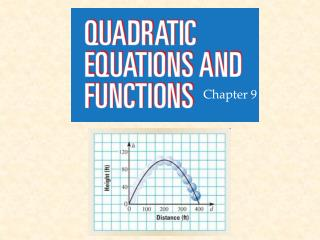DownloadDownload PresentationChapter 9

# Chapter 9

Download Presentation## Chapter 9

- - - - - - - - - - - - - - - - - - - - - - - - - - - E N D - - - - - - - - - - - - - - - - - - - - - - - - - - -
##### Presentation Transcript

1. Chapter 9

2. 9.1 p 507 #23-77 every 3rd one; 97,98

3. 9.1 p 507 #23-77 every 3rd one; 97,98

4. 9.1 p 507 #23-77 every 3rd one; 97,98

5. 9.1 p 507 #23-77 every 3rd one; 97,98

6. 9.1 p 507 #23-77 every 3rd one; 97,98

7. “a” makes the graph open _____ or _____. “a” determines if the parabola is ________ or _______. A positive “b” makes the graph move _________________. http://my.hrw.com/math06_07/nsmedia/tools/Graph_Calculator/graphCalc.html

8. A positive “c” moves the graph ____. A negative “c” moves the graph _______. http://www.classzone.com/vpg_ebooks/ml_algebra_1_2004/resources/assets/9_3_GraphQuadFunc.html http://my.hrw.com/math06_07/nsmedia/tools/Graph_Calculator/graphCalc.html

9. http://my.hrw.com/math06_07/nsmedia/tools/Graph_Calculator/graphCalc.htmlhttp://my.hrw.com/math06_07/nsmedia/tools/Graph_Calculator/graphCalc.html

10. http://my.hrw.com/math06_07/nsmedia/tools/Graph_Calculator/graphCalc.htmlhttp://my.hrw.com/math06_07/nsmedia/tools/Graph_Calculator/graphCalc.html

11. http://my.hrw.com/math06_07/nsmedia/tools/Graph_Calculator/graphCalc.htmlhttp://my.hrw.com/math06_07/nsmedia/tools/Graph_Calculator/graphCalc.html

12. Quadratic function Standard form Parabola Vertex Axis of symmetry http://my.hrw.com/math06_07/nsmedia/tools/Graph_Calculator/graphCalc.html

13. http://my.hrw.com/math06_07/nsmedia/tools/Graph_Calculator/graphCalc.htmlhttp://my.hrw.com/math06_07/nsmedia/tools/Graph_Calculator/graphCalc.html

14. A= Parabola opens: B= C= -b/2a= Axis of symmetry: http://my.hrw.com/math06_07/nsmedia/tools/Graph_Calculator/graphCalc.html

15. A= Parabola opens: B= C= -b/2a= Axis of symmetry: http://my.hrw.com/math06_07/nsmedia/tools/Graph_Calculator/graphCalc.html

16. A= Parabola opens: B= C= -b/2a= Axis of symmetry:

17. A= Parabola opens: B= C= -b/2a= Axis of symmetry: A= Parabola opens: B= C= -b/2a= Axis of symmetry:

18. A= Parabola opens: B= C= -b/2a= Axis of symmetry: http://my.hrw.com/math06_07/nsmedia/tools/Graph_Calculator/graphCalc.html

19. A= Parabola opens: B= C= -b/2a= Axis of symmetry: http://my.hrw.com/math06_07/nsmedia/tools/Graph_Calculator/graphCalc.html

20. A= Parabola opens: B= C= -b/2a= Axis of symmetry: http://my.hrw.com/math06_07/nsmedia/tools/Graph_Calculator/graphCalc.html

21. A= Parabola opens: B= C= -b/2a= Axis of symmetry: http://my.hrw.com/math06_07/nsmedia/tools/Graph_Calculator/graphCalc.html

22. A= Parabola opens: B= C= -b/2a= Axis of symmetry: http://my.hrw.com/math06_07/nsmedia/tools/Graph_Calculator/graphCalc.html

23. A= Parabola opens: B= C= -b/2a= Axis of symmetry: http://my.hrw.com/math06_07/nsmedia/tools/Graph_Calculator/graphCalc.html

24. A= Parabola opens: B= C= -b/2a= Axis of symmetry: http://my.hrw.com/math06_07/nsmedia/tools/Graph_Calculator/graphCalc.html

25. A= Parabola opens: B= C= -b/2a= Axis of symmetry: http://my.hrw.com/math06_07/nsmedia/tools/Graph_Calculator/graphCalc.html

26. A= Parabola opens: B= C= -b/2a= Axis of symmetry: http://my.hrw.com/math06_07/nsmedia/tools/Graph_Calculator/graphCalc.html

27. A= Parabola opens: B= C= -b/2a= Axis of symmetry: http://my.hrw.com/math06_07/nsmedia/tools/Graph_Calculator/graphCalc.html

28. A= Parabola opens: B= C= -b/2a= Axis of symmetry: http://my.hrw.com/math06_07/nsmedia/tools/Graph_Calculator/graphCalc.html

29. A= Parabola opens: B= C= -b/2a= Axis of symmetry: http://my.hrw.com/math06_07/nsmedia/tools/Graph_Calculator/graphCalc.html

30. A= Parabola opens: B= C= -b/2a= Axis of symmetry: http://my.hrw.com/math06_07/nsmedia/tools/Graph_Calculator/graphCalc.html

31. A= -0.006 Parabola opens: down B= 1.2 C=10 -b/2a= -1.2/2(-0.006)=100 Axis of symmetry:100 http://my.hrw.com/math06_07/nsmedia/tools/Graph_Calculator/graphCalc.html

32. http://my.hrw.com/math06_07/nsmedia/tools/Graph_Calculator/graphCalc.htmlhttp://my.hrw.com/math06_07/nsmedia/tools/Graph_Calculator/graphCalc.html

33. A= Parabola opens: B= C= -b/2a= Axis of symmetry: http://my.hrw.com/math06_07/nsmedia/tools/Graph_Calculator/graphCalc.html